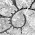## Sunday, March 27, 2016

### Trick to append an object of class `"phylo"` to a `"multiPhylo"` object

I forgot about this trick. Objects of class `"phylo"` and `"multiPhylo"` have S3 combine methods; however when we want to append a object of class `"phylo"` to an object of class `"multiPhylo"` we run into a problem.

So for instance:

``````library(ape)
t1<-rtree(n=20)
t2<-rtree(n=20)
obj<-c(t1,t2)
obj
``````
``````## 2 phylogenetic trees
``````
``````## no problem!
## or
t1<-rmtree(n=20,N=10)
t2<-rmtree(n=100,N=2)
obj<-c(t1,t2)
obj
``````
``````## 12 phylogenetic trees
``````
``````## no problem!
## however:
t1<-rmtree(n=20,N=10)
t2<-rtree(n=20)
obj<-c(t1,t2)
``````
``````## Error in `[<-.multiPhylo`(`*tmp*`, a:N, value = structure(list(edge = structure(c(21L, : at least one element in 'value' is not of class "phylo".
``````
``````## doesn't work
``````

A workaround that is not too hard is to make the `"phylo"` a `"multiPhylo"` object with one tree only, as follows:

``````t2<-list(t2)
class(t2)<-"multiPhylo"
t2
``````
``````## 1 phylogenetic trees
``````
``````obj<-c(t1,t2)
obj
``````
``````## 11 phylogenetic trees
``````
``````## works!
``````

1.This blog post title is a slight rewrite from clickbait fodder. All you needed to do was make it "This One Weird Trick to append an object of class "phylo" to a "multiPhylo" object!"

All joking aside, this trick is very useful...

1.No, I think 'I tried to combine an object of class "multiPhylo" with one of class "phylo" and you won't believe what happened next....'

2.Note: due to the very large amount of spam, all comments are now automatically submitted for moderation.